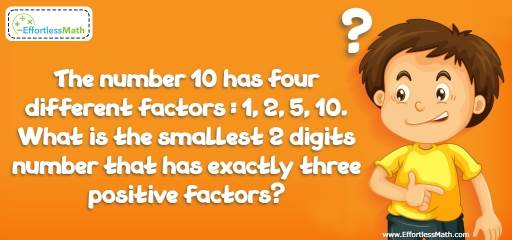# Number Properties Puzzle – Challenge 8

This is a great math challenge about number properties. This math puzzle is a great refresher for those who love mathematics and critical thinking challenges. Let's challenge your brain!## Challenge:

The number 10 has four different factors: 1, 2, 5, 10. What is the smallest 2 digits number that has exactly three positive factors?

### The Absolute Best Book to challenge your Smart Student!

Each prime number has exactly 2 factors, 1 and the number itself. To find the smallest 2 digits number, n, that has exactly three positive factors, we need to find an odd perfect square number. Why?
The factors of number n are 1, n and a prime number. Let’s consider the smallest prime numbers:
2: If 2 is a factor of n, then n should be 4 (1×2×2). This is not the answer. Since, n should be a 2 digits number.
3: If 3 is a factor of n, then n should be 9 (1×3×3). This is not the answer!
5: If 5 is a factor of n, then n should be 25 (1×5×5). Bingo!

### What people say about "Number Properties Puzzle – Challenge 8 - Effortless Math: We Help Students Learn to LOVE Mathematics"?

No one replied yet.

X
30% OFF

Limited time only!

Save Over 30%

SAVE $5 It was$16.99 now it is \$11.99